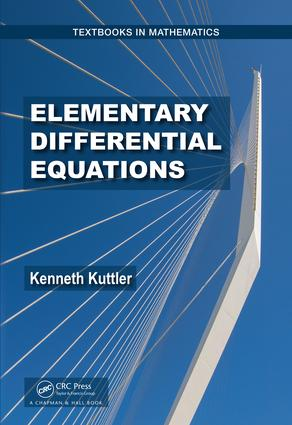# Elementary Differential Equations

## 1st Edition

Chapman and Hall/CRC

574 pages | 89 B/W Illus.

##### Purchasing Options:\$ = USD
Hardback: 9781138740914
pub: 2017-11-08
SAVE ~\$27.00
\$135.00
\$108.00
x
eBook (VitalSource) : 9781315183138
pub: 2017-11-20
from \$67.50

FREE Standard Shipping!

### Description

Elementary Differential Equations presents the standard material in a first course on diﬀerential equations, including all standard methods which have been a part of the subject since the time of Newton and the Bernoulli brothers. The emphasis in this book is on theory and methods and diﬀerential equations as a part of analysis.

Diﬀerential equations is worth studying, rather than merely some recipes to be used in physical science. The text gives substantial emphasis to methods which are generally presented ﬁrst with theoretical considerations following. Essentially all proofs of the theorems used are included, making the book more useful as a reference.

The book mentions the main computer algebra systems, yet the emphasis is placed on MATLAB and numerical methods which include graphing the solutions and obtaining tables of values.

Featured applications are easily understood. Complete explanations of the mathematics and emphasis on methods for ﬁnding solutions are included.

### Table of Contents

Preface; 1 Some Prerequisite Topics; I First Order Scalar Differential Equations, Methods; 2 The Idea Of A Differential Equation; 3 Homogeneous Linear Equations; 4 Nonhomogeneous Equations; 5 Laplace Transform Methods; III Series Methods For Scalar; 6 Power Series; 7 Power Series Methods; IV First Order Linear Systems; 8 First Order Systems Of Differential Equations, Theory; 9 Methods For First Order Systems; V Partial Differential Equations; 10 Boundary Value Problems And Fourier Series; 11 Some Famous Partial Differential Equations; VI Non-linear Systems Of O.D.E.; 12 Theory Of Ordinary Differential Equations; 13 Equilibrium Points And Limit Cycles; 14 The Center Manifold; Appendix A: Calculus Review 381; Appendix B Series, Appendix C Review Of Linear Algebra; Appendix D Theory Of Functions Of Many Variables; Appendix E Spectral Theory; Appendix F Linear Transformations; Appendix G Implicit Function Theorem; Appendix H The Jordan Curve Theorem; Bibliography; Index

### About the Author

Kenneth Kutler is Professor, Brigham Young University, holds his PhD from University of Texas.His primary area of research is Partial Differential Equations and Inclusions.

### Subject Categories

##### BISAC Subject Codes/Headings:
MAT003000
MATHEMATICS / Applied
MAT007000
MATHEMATICS / Differential Equations